HomeCompositio Mathematica

# Spinor groups with good reduction

Published online by Cambridge University Press:  07 March 2019

Corresponding

## Abstract

Let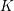$K$ be a two-dimensional global field of characteristic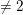$\neq 2$ and let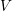$V$ be a divisorial set of places of$K$ . We show that for a given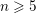$n\geqslant 5$ , the set of$K$ -isomorphism classes of spinor groups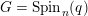$G=\operatorname{Spin}_{n}(q)$ of nondegenerate$n$ -dimensional quadratic forms over$K$ that have good reduction at all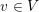$v\in V$ is finite. This result yields some other finiteness properties, such as the finiteness of the genus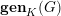$\mathbf{gen}_{K}(G)$ and the properness of the global-to-local map in Galois cohomology. The proof relies on the finiteness of the unramified cohomology groups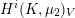$H^{i}(K,\unicode[STIX]{x1D707}_{2})_{V}$ for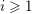$i\geqslant 1$ established in the paper. The results for spinor groups are then extended to some unitary groups and to groups of type$\mathsf{G}_{2}$ .

## MSC classification

Type
Research Article
Information
Compositio Mathematica , March 2019 , pp. 484 - 527

## Access options

Get access to the full version of this content by using one of the access options below.

## Footnotes

The first author was supported by the Canada Research Chairs Program and by an NSERC research grant. The second author was partially supported by the Simons Foundation. During the preparation of the final version of the paper, he visited Princeton University and the Institute for Advanced Study, and would like to thankfully acknowledge the hospitality of both institutions. The third author was partially supported by an AMS-Simons Travel Grant. We are grateful to P. Gille and M. Rapoport for useful conversations. Last but not least, we would like to express our gratitude to all anonymous referees whose comments, insights and suggestions helped to improve the original paper significantly.

## References

Berhuy, G., Finiteness of R-equivalence groups of some adjoint classical groups of type 2 D 3 , J. Algebra 309 (2007), 360366.CrossRefGoogle Scholar
Berhuy, G., An introduction to Galois cohomology and its applications, London Mathematical Society Lecture Note Series, vol. 377 (Cambridge University Press, Cambridge, 2010).CrossRefGoogle Scholar
Bloch, S. and Ogus, A., Gersten’s conjecture and the homology of schemes , Ann. Sci. Éc. Norm. Supér. (4) 7 (1974), 181201.CrossRefGoogle Scholar
Brussel, E., On Saltman’s p-adic curves papers , in Quadratic forms, linear algebraic groups, and cohomology, Developments in Mathematics, vol. 18 (Springer, New York, 2010), 1339.CrossRefGoogle Scholar
Cassels, J. W. S. and Fröhlich, A. (eds), Algebraic number theory, second edition (London Mathematical Society, London, 2010).Google Scholar
Chernousov, V. I., Gille, P. and Pianzola, A., Torsors over the punctured affine line , Amer. J. Math. 134 (2012), 15411583.CrossRefGoogle Scholar
Chernousov, V. I., Neher, E., Pianzola, A. and Yahorau, U., On conjugacy of Cartan subalgebras in extended affine Lie algebras , Adv. Math. 290 (2016), 260292.CrossRefGoogle Scholar
Chernousov, V. I., Rapinchuk, A. S. and Rapinchuk, I. A., The genus of a division algebra and the unramified Brauer group , Bull. Math. Sci. 3 (2013), 211240.CrossRefGoogle Scholar
Chernousov, V. I., Rapinchuk, A. S. and Rapinchuk, I. A., Division algebras with the same maximal subfields , Russian Math. Surveys 70 (2015), 91122.CrossRefGoogle Scholar
Chernousov, V. I., Rapinchuk, A. S. and Rapinchuk, I. A., On the size of the genus of a division algebra , Proc. Steklov Inst. Math. 292 (2016), 6393.CrossRefGoogle Scholar
Chernousov, V. I., Rapinchuk, A. S. and Rapinchuk, I. A., On some finiteness properties of algebraic groups over finitely generated fields , C. R. Math. Acad. Sci. Paris, Ser. I 354 (2016), 869873.CrossRefGoogle Scholar
Chernousov, V. I., Rapinchuk, A. S. and Rapinchuk, I. A., The finiteness of the genus of a finite-dimensional division algebras, and some generalizations, Israel J. Math., to appear.Google Scholar
Chernousov, V. I., Rapinchuk, A. S. and Rapinchuk, I. A., Algebraic groups with the same tori, weakly commensurable Zariski-dense subgroups, and good reduction, in preparation.Google Scholar
Colliot-Thélène, J.-L., Birational invariants, purity and the Gersten conjecture , in K-theory and algebraic geometry: connections with quadratic forms and division algebras (Santa Barbara, CA, 1992), Proceedings of Symposia in Pure Mathematics, vol. 58 (American Mathematical Society, Providence, RI, 1995), 164.Google Scholar
Colliot-Thélène, J.-L., Quelques résultats de finitude pour le groupe SK 1 d’une algèbre de biquaternions , J. K-Theory 10 (1996), 3148.CrossRefGoogle Scholar
Colliot-Thélène, J.-L., Hoobler, R. and Kahn, B., The Bloch–Ogus–Gabber theorem , in Algebraic K-theory (Toronto, ON, 1996), Fields Institute Communications, vol. 16 (American Mathematical Society, Providence, RI, 1997), 3194.Google Scholar
Colliot-Thélène, J.-L., Parimala, R. and Suresh, V., Patching and local-global principles for homogeneous spaces over function fields of p-adic curves , Comment. Math. Helv. 87 (2012), 10111033.CrossRefGoogle Scholar
Colliot-Thélène, J.-L. and Sansuc, J.-J., Fibrés quadratiques et composantes connexes réelles , Math. Ann. 244 (1979), 105134.CrossRefGoogle Scholar
Colliot-Thélène, J.-L., Sansuc, J.-J. and Soulé, C., Torsion dans le groupe de Chow de codimension deux , Duke Math. J. 50 (1983), 763801.CrossRefGoogle Scholar
Conrad, B., Non-split reductive groups over, in Autour des schémas en groupes, Vol. II, Panoramas et Synthèses, vol. 46 (Soc. Math. France, Paris, 2015), 193253.Google Scholar
Cutkosky, S. D., Introduction to Algebraic Geometry, Graduate Studies in Mathematics, vol. 188 (American Mathematical Society, Providence, RI, 2018).CrossRefGoogle Scholar
Faltings, G., Endlichkeitssätze für abelsche Varietäten über Zahlkörpern , Invent. Math. 73 (1983), 349366.CrossRefGoogle Scholar
Garibaldi, S., Merkurjev, A. and Serre, J.-P., Cohomological invariants in Galois cohomology, University Lecture Series, vol. 28 (American Mathematical Society, Providence, RI, 2003).CrossRefGoogle Scholar
Gerstein, L. J., Basic quadratic forms, Graduate Studies in Mathematics, vol. 90 (American Mathematical Society, Providence, RI, 2008).CrossRefGoogle Scholar
Gille, P. and Szamuely, T., Central simple algebras and Galois cohomology (Cambridge University Press, Cambridge, 2006).CrossRefGoogle Scholar
Gross, B. H., Groups over, Invent. Math. 124 (1996), 263279.CrossRefGoogle Scholar
Harder, G., Halbeifache Gruppenschemata über Dedekindringen , Invent. Math. 4 (1967), 165191.CrossRefGoogle Scholar
Hartshorne, R., Algebraic geometry, Geometry & Topology Monographs, vol. 52 (Springer, Berlin, 1977).CrossRefGoogle Scholar
Jannsen, U., Principe de Hasse cohomologique , in Séminaire de Théorie des Nombres (Soc. Math. France, Paris, 1989–90), 121140.Google Scholar
Jannsen, U., Hasse principles for higher-dimensional fields , Ann. of Math. (2) 183 (2016), 171.CrossRefGoogle Scholar
Jannsen, U., Sato, S. and Saito, K., Étale duality for constructible sheaves on arithmetic schemes , J. reine angew. Math. 688 (2014), 165.CrossRefGoogle Scholar
Javanpeykar, A. and Loughran, D., Good reduction of algebraic groups and flag varieties , Arch. Math. 104 (2015), 133143.CrossRefGoogle Scholar
Kahn, B., Sur le groupe des clsses d’un schéma arithmétiques , Bull. Soc. Math. France 134 (2006), 395415.CrossRefGoogle Scholar
Kato, K., A Hasse principle for two dimensional global fields , J. reine angew. Math. 366 (1986), 142183, with an appendix by J.-L. Colliot-Thélène.Google Scholar
Knus, M.-A., Merkurjev, A., Rost, M. and Tignol, J.-P., The book of involutions, Colloquium Publications, vol. 44 (American Mathematical Society, Providence, RI, 1998).CrossRefGoogle Scholar
Lam, T. Y., Introduction to quadratic forms over fields, Graduate Studies in Mathematics, vol. 67 (American Mathematical Society, Providence, RI, 2005).Google Scholar
Merkurjev, A. S. and Suslin, A. A., K-cohomology of Severi-Brauer varieties and the norm residue homomorphism , Izv. Akad. Nauk SSSR Ser. Mat. 46 (1982), 10111046.Google Scholar
Milne, J. S., Étale cohomology (Princeton University Press, Princeton, NJ, 1980).Google Scholar
Milnor, J., Algebraic K-theory and quadratic forms , Invent. Math. 9 (1970), 318344.CrossRefGoogle Scholar
Orlov, D., Vishik, A. and Voevodsky, V., An exact sequence for KM /2 with applications to quadratic forms , Ann. of Math. (2) 165 (2007), 113.CrossRefGoogle Scholar
Parimala, R., A Hasse principle for quadratic forms over function fields , Bull. Amer. Math. Soc. (N.S.) 51 (2014), 447461.CrossRefGoogle Scholar
Parimala, R. and Suresh, V., Isotropy of quadratic forms over function fields of p-adic curves , Publ. Math. Inst. Hautes Études Sci. 88 (1998), 129150.CrossRefGoogle Scholar
Parimala, R. and Suresh, V., The u-invariant of the function fields of p-adic curves , Ann. of Math. (2) 172 (2010), 13911405.CrossRefGoogle Scholar
Platonov, V. P. and Rapinchuk, A. S., Algebraic groups and number theory (Academic Press, New York, 1994).Google Scholar
Prasad, G. and Rapinchuk, A. S., Existence of irreducible-regular elements in Zariski-dense subgroups , Math. Res. Lett. 10 (2003), 2132.CrossRefGoogle Scholar
Prasad, G. and Rapinchuk, A. S., Weakly commensurable arithmetic groups and isospectral locally symmetric spaces , Publ. Math. Inst. Hautes Études Sci. 109 (2009), 113184.CrossRefGoogle Scholar
Prasad, G. and Rapinchuk, A. S., Weakly commensurable groups, with applications to differential geometry , in Handbook of Group Actions, Vol. I, Advanced Lectures in Mathematics, vol. 32 (International Press, Somerville, MA, 2015), 495524.Google Scholar
Prasad, G. and Rapinchuk, A. S., Generic elements of a Zariski-dense subgroup form an open subset , Trans. Moscow Math. Soc. 78 (2017), 299314.CrossRefGoogle Scholar
Raghunathan, M. S. and Ramanathan, A., Principal bundles on the affine line , Proc. Indian Acad. Sci. Math. Sci. 93 (1984), 137145.CrossRefGoogle Scholar
Rapinchuk, A. S., Towards the eigenvalue rigidity of Zariski-dense subgroups , in Proceedings of the international congress of mathematicians (Seoul 2014), Vol. II (Kyung Moon Sa, Seoul, 2014), 247269.Google Scholar
Rapinchuk, A. S. and Rapinchuk, I. A., On division algebras having the same maximal subfields , Manuscripta Math. 132 (2010), 273293.CrossRefGoogle Scholar
Rapinchuk, I. A., A generalization of Serre’s condition (F) with applications to the finiteness of unramified cohomology , Math. Z. (2018), doi:10.1007/s00209-018-2079-0.Google Scholar
Rapinchuk, I. A., On residue maps for affine curves , J. Pure Appl. Algebra 223 (2019), 965975.CrossRefGoogle Scholar
Samuel, P., A propos du théorèm des unités , Bull. Sci. Math. (2) 90 (1966), 8996.Google Scholar
SGA 1: Revêtements étales et groupe fondamentale, Lecture Notes in Mathematics, vol. 224 (Springer, Berlin, 1971).Google Scholar
Artin, M., Deligne, P., Grothendieck, A. and Verdier, J.-L., SGA 4, tome 3, Lecture Notes in Mathematics, vol. 305 (Springer, New York, 1973).Google Scholar
Deligne, P., Cohomologie étale , in SGA 4½, Lecture Notes in Mathematics, vol. 569 (Springer, 1977).Google Scholar
Serre, J.-P., Galois cohomology (Springer, Berlin, 1997).CrossRefGoogle Scholar
The Stacks Project (2019) Étale cohomology. https://stacks.math.columbia.edu/tag/03N1.Google Scholar
Voevodsky, V., Motivic cohomology with/2-coefficients , Publ. Math. Inst. Hautes Études Sci. 98 (2003), 59104.CrossRefGoogle Scholar
Voevodsky, V., On motivic cohomology with/-coefficients , Ann. of Math. (2) 174 (2011), 401438.CrossRefGoogle Scholar

### Full text views

Full text views reflects PDF downloads, PDFs sent to Google Drive, Dropbox and Kindle and HTML full text views.

Total number of HTML views: 3
Total number of PDF views: 98 *
View data table for this chart

* Views captured on Cambridge Core between 07th March 2019 - 1st December 2020. This data will be updated every 24 hours.

Hostname: page-component-6d4bddd689-7dcxl Total loading time: 15.676 Render date: 2020-12-01T09:32:38.379Z Query parameters: { "hasAccess": "0", "openAccess": "0", "isLogged": "0", "lang": "en" } Feature Flags last update: Tue Dec 01 2020 08:42:57 GMT+0000 (Coordinated Universal Time) Feature Flags: { "metrics": true, "metricsAbstractViews": false, "peerReview": true, "crossMark": true, "comments": true, "relatedCommentaries": true, "subject": true, "clr": false, "languageSwitch": true }

# Send article to Kindle

Note you can select to send to either the @free.kindle.com or @kindle.com variations. ‘@free.kindle.com’ emails are free but can only be sent to your device when it is connected to wi-fi. ‘@kindle.com’ emails can be delivered even when you are not connected to wi-fi, but note that service fees apply.

Find out more about the Kindle Personal Document Service.

Spinor groups with good reduction
Available formats
×

# Send article to Dropbox

To send this article to your Dropbox account, please select one or more formats and confirm that you agree to abide by our usage policies. If this is the first time you use this feature, you will be asked to authorise Cambridge Core to connect with your <service> account. Find out more about sending content to Dropbox.

Spinor groups with good reduction
Available formats
×

# Send article to Google Drive

To send this article to your Google Drive account, please select one or more formats and confirm that you agree to abide by our usage policies. If this is the first time you use this feature, you will be asked to authorise Cambridge Core to connect with your <service> account. Find out more about sending content to Google Drive.

Spinor groups with good reduction
Available formats
×
×